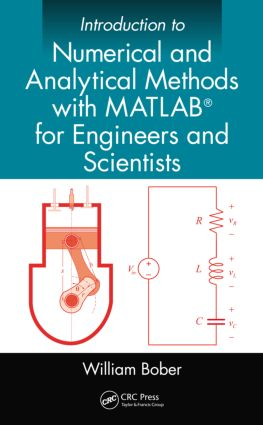# Introduction to Numerical and Analytical Methods with MATLAB® for Engineers and Scientists

## 1st Edition

CRC Press

556 pages | 208 B/W Illus.

Hardback: 9781466576025
pub: 2013-11-12
SAVE ~\$30.00
\$150.00
\$120.00
x
eBook (VitalSource) : 9780429101618
pub: 2013-11-12
from \$75.00

FREE Standard Shipping!

### Description

Introduction to Numerical and Analytical Methods with MATLAB® for Engineers and Scientists provides the basic concepts of programming in MATLAB for engineering applications.

• Teaches engineering students how to write computer programs on the MATLAB platform

• Examines the selection and use of numerical and analytical methods through examples and case studies

• Demonstrates mathematical concepts that can be used to help solve engineering problems, including matrices, roots of equations, integration, ordinary differential equations, curve fitting, algebraic linear equations, and more

The text covers useful numerical methods, including interpolation, Simpson’s rule on integration, the Gauss elimination method for solving systems of linear algebraic equations, the Runge-Kutta method for solving ordinary differential equations, and the search method in combination with the bisection method for obtaining the roots of transcendental and polynomial equations. It also highlights MATLAB’s built-in functions. These include interp1 function, the quad and dblquad functions, the inv function, the ode45 function, the fzero function, and many others. The second half of the text covers more advanced topics, including the iteration method for solving pipe flow problems, the Hardy-Cross method for solving flow rates in a pipe network, separation of variables for solving partial differential equations, and the use of Laplace transforms to solve both ordinary and partial differential equations.

This book serves as a textbook for a first course in numerical methods using MATLAB to solve problems in mechanical, civil, aeronautical, and electrical engineering. It can also be used as a textbook or as a reference book in higher level courses.

### Reviews

"…provides in a very simple and clear way the first skills in computer programming under the MATLAB platform. The author uses various mathematical concepts (matrices, roots of equations, integration, ordinary differential equations, interpolation, etc.) in order to solve mathematical problems associated with engineering type problems. …the graphic of the work is fairly suggestive and assists a smooth learning."

Zentralblatt MATH 1284

Computer Programming with MATLAB® for Engineers

Introduction

Computer Usage in Engineering

Mathematical Model

Computer Programming

Why MATLAB®?

MATLAB® Programming Language

Building Blocks in Writing a Program

Conventions in This Book

MATLAB® Fundamentals

Introduction

MATLAB® Desktop

Constructing a Program in MATLAB®

MATLAB® Fundamentals

MATLAB® Input/Output

Loops

MATLAB® Graphics

Conditional Operators and Alternate Paths

Working with Built-In Functions with Vector Arguments

More on MATLAB® Graphics

Debugging a Program

Projects

References

Taylor Series, Self-Written Functions and MATLAB®’s interp1 Function

Introduction

Functions Expressed as a Series

Self-Written Functions

Anonymous Functions

MATLAB®’s interp1 Function

Working with Characters and Strings

Projects

References

Matrices

Introduction

Matrix Operations

System of Linear Equations

Statics Truss Problem

Resistive Circuit Problem

Gauss Elimination

Gauss–Jordan Method

Number of Solutions

Inverse Matrix

Eigenvalue Problem in Mechanical Vibrations

Eigenvalue Problem in Electrical Circuits

Projects

Reference

Roots of Algebraic and Transcendental Equations

Introduction

The Search Method

Bisection Method

Newton–Raphson Method

MATLAB®’s Root-finding Functions

Projects

Reference

Numerical Integration

Introduction

Numerical Integration with the Trapezoidal Rule

Numerical Integration and Simpson’s Rule

Projects

Numerical Integration of Ordinary Differential Equations

Introduction

Initial Value Problem

Euler Algorithm

Modified Euler Method with Predictor–Corrector Algorithm

Fourth-Order Runge–Kutta Method

System of Two First-Order Differential Equations

Single Second-Order Equation

MATLAB®’s ODE Function

Projects

Boundary Value Problems of Ordinary Differential Equations

Introduction

Difference Formulas

Solution of a Tri-Diagonal System of Linear Equations

Projects

Curve Fitting

Introduction

Method of Least Squares

Curve Fitting with the Exponential Function

MATLAB®’s Curve Fitting Functions

Cubic Splines

MATLAB®’s Cubic Spline Curve Fitting Function

Curve Fitting with Fourier Series

Projects

Introduction

Typical Building Blocks in Constructing a Model

Tips for Constructing and Running Models

Constructing a Subsystem

Using the Mux and Fcn Blocks

Using the Transfer Fcn Block

Using the Relay and Switch Blocks

Trigonometric Function Blocks

To Workspace Block

Projects

Reference

Optimization

Introduction

Unconstrained Optimization Problems

Method of Steepest Descent

MATLAB®’s fminbnd and fminsearch Functions

Optimization with Constraints

Lagrange Multipliers

MATLAB®’s fmincon Function

Projects

Reference

Iteration Method

Introduction

Iteration in Pipe Flow Analysis

Hardy–Cross Method

Projects

References

Partial Differential Equations

Classification of Partial Differential Equations

Solution by Separation of Variables

Review of Finite-Difference Formulas

Finite-Difference Methods Applied to Partial Differential

Equations

The Gauss–Seidel Method

Projects

Laplace Transforms

Introduction

Laplace Transform and Inverse Transform

Transforms of Derivatives

Ordinary Differential Equations, Initial Value Problem

MATLAB®’s residue Function

Unit Step Function

Convolution

Laplace Transforms Applied to Circuits

Delta Function

Laplace Transforms Applied to Partial Differential Equations

Projects

References

Appendices

Index

William Bober, PhD, received his BS in civil engineering from the City College of New York (CCNY), his MS in engineering science from Pratt Institute, and his PhD in engineering science and aerospace engineering from Purdue University.

He worked as an associate engineering physicist in the Applied Mechanics Department at Cornell Aeronautical Laboratory in Buffalo, New York, was employed as an associate professor in the Department of Mechanical Engineering at the Rochester Institute of Technology, and is an associate professor at Florida Atlantic University (FAU), working in the Department of Mechanical Engineering and the Department of Civil Engineering at FAU.